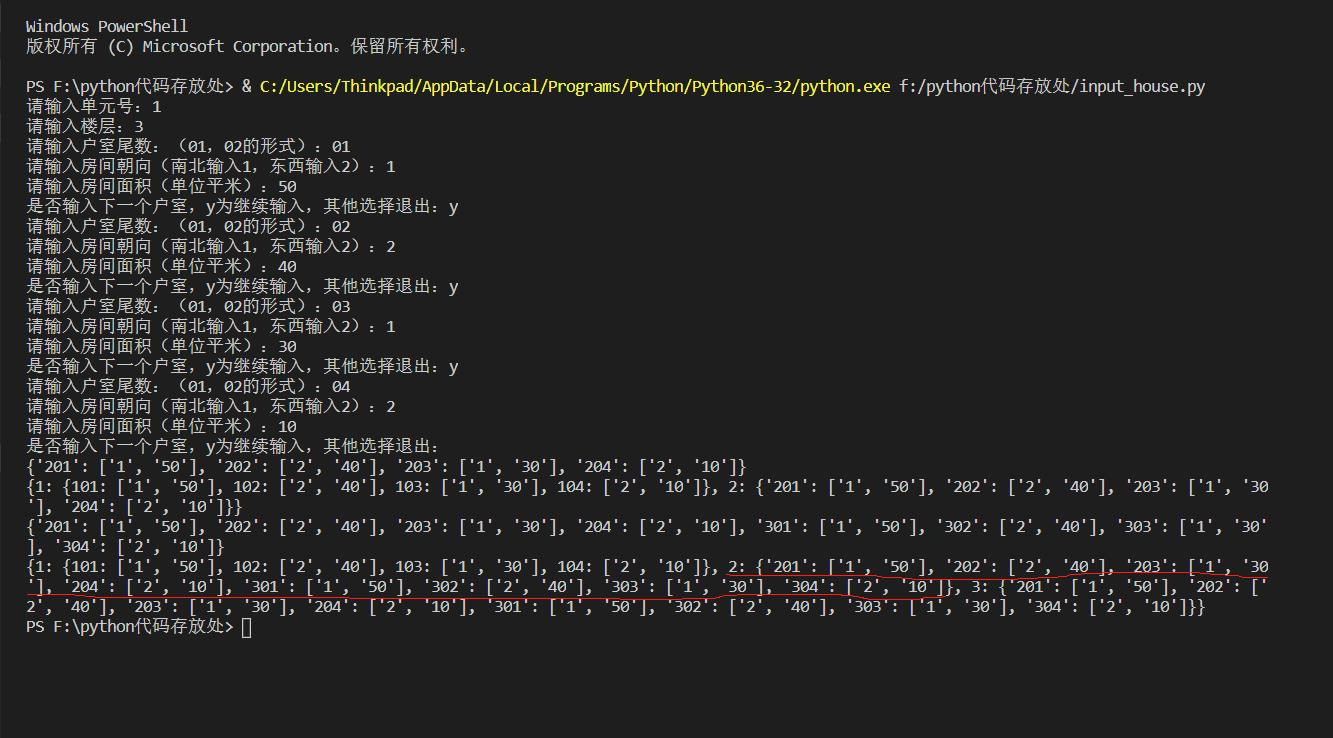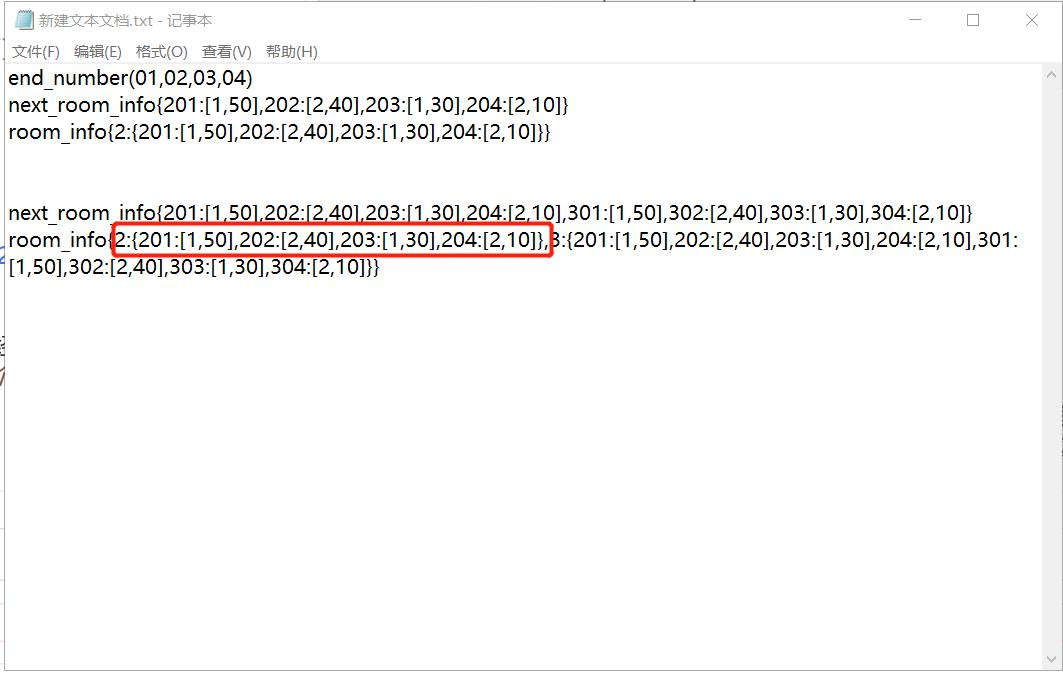2020-01-03 21:42

# 麻烦问下我的这段代码为什么运行结果跟我想的不一样，求指导？

``````unit=input('请输入单元号：')
floor=int(input('请输入楼层：'))
end_number=[]
first_room_info={}
while True:
room_end_input=input('请输入户室尾数：（01，02的形式）：')
end_number.append(room_end_input)
room_number=int('1'+room_end_input)
direction=input('请输入房间朝向（南北输入1，东西输入2）：')
area=input('请输入房间面积（单位平米）：')
first_room_info[room_number]=[direction,area]
choice=input('是否输入下一个户室，y为继续输入，其他选择退出：')
if choice=='y':
pass
else:
break
room_info={}
next_room_info={}
room_info=first_room_info
for a in range(2,floor+1):
for i in range(len(end_number)):
number=str(a)+end_number[i]
info=first_room_info[int('1'+end_number[i])]
next_room_info[number]=info
room_info[a]=next_room_info
print(next_room_info)
print(room_info)
``````• 写回答
• 好问题 提建议
• 关注问题
• 收藏
• 邀请回答